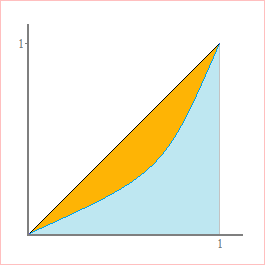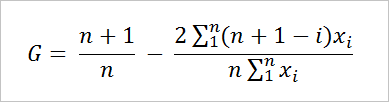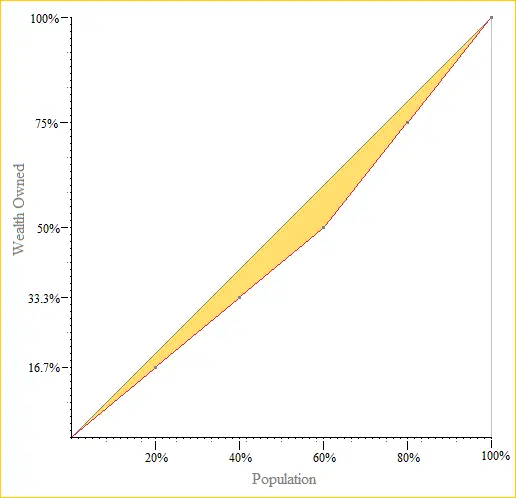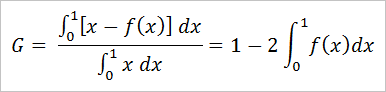# Gini Coefficient Calculator

 Gini Coeff. Calculator: Enter Data Below Gini Coefficient =

The Lorenz curve is the cumulative distribution function of wealth when individual's financial holdings are ordered from least to greatest. Each (x, y) point on the curve means that the bottom x% of the population owns y% of the wealth.

The Gini coefficient, named after Italian statistician Corrado Gini, is a dimensionless measure of statistical dispersion that is frequently used in the analysis of income distribution.

The Gini coefficient, G, of a data set or income distribution curve ranges from 0 to 1, with 1 being the most unequal distribution of wealth (one person owns everything) and 0 being the most equal (each person owns an equal share).

Mathematically, G is the ratio of the area between the wealth distribution curve (Lorenz curve) and the line of equal distribution (straight line) to the area under the equal distribution curve. This is best described graphically:### Computing the Gini Coefficient (Empirical Distribution)

For an empirical Lorenz curve, one generated by discrete data points, you can compute the Gini coefficient with the formulawhere the xi are ordered from least to greatest. For example, suppose \$12 is distributed among five people as follows: two people receive \$3 and three people receive \$2. In this scenario,

the bottom 20% own \$2, or 16.7% of the wealth
the bottom 40% own \$4, or 33.3% of the wealth
the bottom 60% own \$6, or 50% of the wealth
the bottom 80% own \$9, or 75% of the wealth
the bottom 100% own \$12, or 100% of the wealth

The Lorenz curve isand the Gini coefficient is

6/5 - (2*33)/(5*12) = 1.2 -1.1 = 0.1

### Computing the Gini Coefficient (Theoretic Distribution)

If the Lorenz curve is given by a function f(x) over the interval [0, 1], then the Gini coefficient can be found with integration. The formula isFor example, if the Lorenz curve is g(z) = 0.25z4z, then the Gini coefficient is 0.33782.